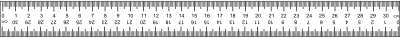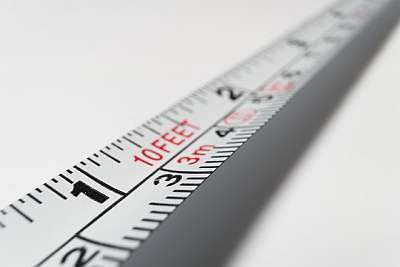# Meters to Inches Calculator

Instructions: Use this calculator to convert a meters to inches, showing all the steps. Please type the number of meters you would like to convert in the form below:Type the number of meters:

## Using this Meter to Inches Calculator

This metric converter allows to conduct a simple operation, which is to convert a length in meters to the equivalent length in inches.

Why you would like to conduct such conversion? Because it provides a bridge between two different standards: the metric unit system and the imperial unit system.

Normally, reports are written consistently in either metric or imperial notation, and from time to time we will need to pass from one system to the other, and then we will typically have to convert all the numbers from one system to the other.## How do you convert meters to inches

The strategy is simple, and you need to use direct proportionality. In fact, the conversion formula for meters and inches is very simply deduced if you are able to apply direct proportionality.

### Steps for the conversion of meters to inches

1. First, notice that 1 meter is the same as 39.37 inches. This is the basic starting point
2. Assume you have M meters. You want to know many inches I will be equivalent to M meters
3. The corresponding proportion you need to solve is $$\displaystyle \frac{1}{39.37} = \frac{M}{I}$$
4. Solving the above proportion leads to the following conversion formula: $$I = 39.37 \cdot M$$### Examples: Converting meters to inches

What is 3.2 meters in inches?

Solution: According to the steps that have been laid out, first we identify the given number of meters we want to convert, which in this case is $$M = 3.2$$ meters. The formula for expressing this length in meters to inches is

$I = 39.37 M$

Plugging the value M = 3.2 into the equation

$I = 39.37 \times 3.2 = 125.984 \text{ inches}$

## Other metric conversion calculators

As we mention before, the metric and imperial systems are different, using different basic units (meters and centimeters for the metric system, and with feet and inches for the imperial system).

Although the calculation is easy, from time to time you will need to do the conversions and having a calculator to do the work can come in handy.

So then, depending on what you want, you can use our meters to feet, centimeters to inches, centimeters to feet, imperial to meters or imperial to centimeters. calculators, based on your requirements.

Possible you won't memorize all the required formulas, to do all the conversions, but you should be able to retain the idea of proportionality, starting with a basic equivalence, and converting from there.